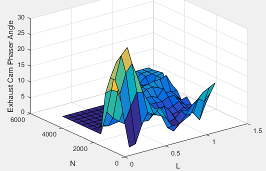## Engine Calibration Maps

Calibration maps are a key part of the engine plant and controller models available in the Powertrain Blockset™. Engine models use the maps to represent engine behavior and to store optimal control parameters. Using calibration maps in control design leads to flexible, efficient control algorithms and estimators that are suitable for electronic control unit (ECU) implementation.

To develop the calibration maps for engine plant and controller models in the reference applications, MathWorks® developed and used processes to measure performance data from 1.5–L spark-ignition (SI) and compression-ignition (CI) engine models provided by Gamma Technologies LLC.

To represent the behavior of engine plants and controllers specific to your application, you can develop your own engine calibration maps. The data required for calibration typically comes from engine dynamometer tests or engine hardware design models.

### Engine Plant Calibration Maps

The engine plant model calibration maps in the Powertrain Blockset SI and CI reference applications affect the engine response to control inputs (for example, spark timing, throttle position, and cam phasing).

To develop the calibration maps in the Powertrain Blockset engine plant models, MathWorks used GT-POWER models from the GT-SUITE modeling library in a Simulink®-based virtual dynamometer. MathWorks used the Model-Based Calibration Toolbox™ to create design-of-experiment (DoE) test plans. The Simulink-based virtual dynamometer executed the DoE test plan on GT-POWER 1.5–L SI and CI reference engines. MathWorks used the Model-Based Calibration Toolbox to develop the engine plant model calibration maps from the GT-POWER.

### Engine Controller Calibration Maps

The engine controller model calibration maps in the reference applications represent the optimal open-loop control commands for given engine operating points.

To develop the calibration maps for the SI engine controller, MathWorks used the GT-POWER reference engine models in a virtual engine calibration optimization (VECO) process. The process optimized the open-loop control commands for 1.5–L SI engine, subject to engine operating constraints for knock, turbocharger speed, and exhaust temperature.

To develop the calibration maps for the CI engine controller, MathWorks used the DOE test data from the GT-POWER 1.5–L CI reference model operated at minimum brake-specific fuel consumption (BSFC).

### Calibration Maps in Compression-Ignition (CI) Blocks

In the engine models, the Powertrain Blockset blocks implement these calibration maps.

MapUsed ForInDescription

Volumetric efficiency

CI Controller

The volumetric efficiency lookup table is a function of the intake manifold absolute pressure at intake valve closing (IVC) and engine speed

`${\eta }_{v}={f}_{{\eta }_{v}}\left(MAP,N\right)$`

where:

• ${\eta }_{v}$ is engine volumetric efficiency, dimensionless.

• MAP is intake manifold absolute pressure, in KPa.

• N is engine speed, in rpm.Optimal main start of injection (SOI) timing

CI Engine Torque Structure Model

CI Controller

The optimal main start of injection (SOI) timing lookup table, ƒSOIc, is a function of the engine speed and injected fuel mass, SOIc = ƒSOIc(F,N), where:

• SOIc is optimal SOI timing, in degATDC.

• F is compression stroke injected fuel mass, in mg per injection.

• N is engine speed, in rpm.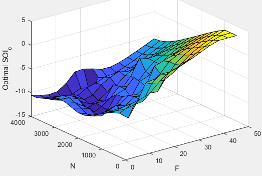Optimal intake manifold gas pressure

CI Engine Torque Structure Model

CI Controller

The optimal intake manifold gas pressure lookup table, ƒMAP, is a function of the engine speed and injected fuel mass, MAP = ƒMAP(F,N), where:

• MAP is optimal intake manifold gas pressure, in Pa.

• F is compression stroke injected fuel mass, in mg per injection.

• N is engine speed, in rpm.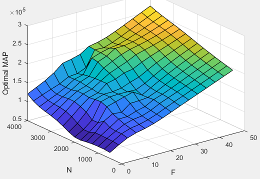Optimal exhaust manifold gas pressure

CI Controller

The optimal exhaust manifold gas pressure lookup table, ƒEMAP, is a function of the engine speed and injected fuel mass, EMAP = ƒEMAP(F,N), where:

• EMAP is optimal exhaust manifold gas pressure, in Pa.

• F is compression stroke injected fuel mass, in mg per injection.

• N is engine speed, in rpm.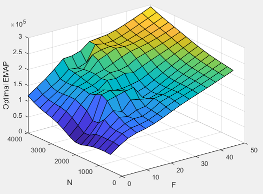Optimal intake manifold gas temperature

CI Controller

The optimal intake manifold gas temperature lookup table, ƒMAT, is a function of the engine speed and injected fuel mass, MAT = ƒMAT(F,N), where:

• MAT is optimal intake manifold gas temperature, in K.

• F is compression stroke injected fuel mass, in mg per injection.

• N is engine speed, in rpm.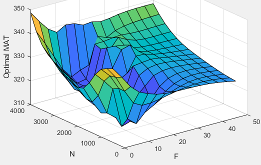Optimal intake gas oxygen percent

CI Controller

The optimal intake gas oxygen percent lookup table, ƒO2, is a function of the engine speed and injected fuel mass, O2PCT = ƒO2(F,N), where:

• O2PCT is optimal intake gas oxygen, in percent.

• F is compression stroke injected fuel mass, in mg per injection.

• N is engine speed, in rpm.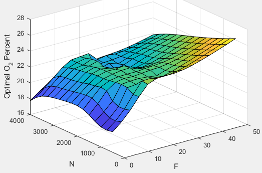Optimal fuel rail pressure

CI Engine Torque Structure Model

CI Controller

The optimal fuel rail pressure lookup table, ƒfuelp, is a function of the engine speed and injected fuel mass, FUELP = ƒfuelp(F,N), where:

• FUELP is optimal fuel rail pressure, in MPa.

• F is compression stroke injected fuel mass, in mg per injection.

• N is engine speed, in rpm.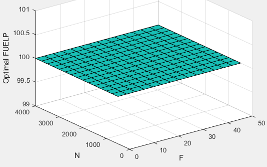Optimal gross indicated mean effective pressure

CI Engine Torque Structure Model

CI Controller

The optimal gross indicated mean effective pressure lookup table, ƒimepg, is a function of the engine speed and injected fuel mass, IMEPG = ƒimepg(F,N), where:

• IMEPG is optimal gross indicated mean effective pressure, in Pa.

• F is compression stroke injected fuel mass, in mg per injection.

• N is engine speed, in rpm.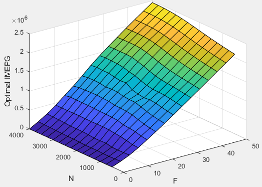Optimal friction mean effective pressure

CI Engine Torque Structure Model

CI Controller

The optimal friction mean effective pressure lookup table, ƒfmep, is a function of the engine speed and injected fuel mass, FMEP = ƒfmep(F,N), where:

• FMEP is optimal friction mean effective pressure, in Pa.

• F is compression stroke injected fuel mass, in mg per injection.

• N is engine speed, in rpm.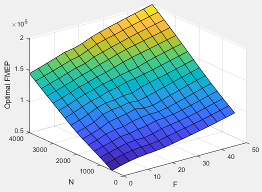Optimal pumping mean effective pressure

CI Engine Torque Structure Model

CI Controller

The optimal pumping mean effective pressure lookup table, ƒpmep, is a function of the engine speed and injected fuel mass, PMEP = ƒpmep(F,N), where:

• PMEP is optimal pumping mean effective pressure, in Pa.

• F is compression stroke injected fuel mass, in mg per injection.

• N is engine speed, in rpm.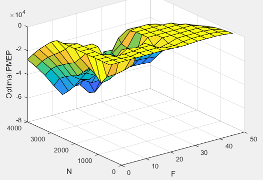Main SOI timing efficiency multiplier

CI Controller

The main start of injection (SOI) timing efficiency multiplier lookup table, ƒSOIeff, is a function of the engine speed and main SOI timing relative to optimal timing, SOIeff = ƒSOIeff(ΔSOI,N), where:

• SOIeff is main SOI timing efficiency multiplier, dimensionless.

• ΔSOI is main SOI timing relative to optimal timing, in degBTDC.

• N is engine speed, in rpm.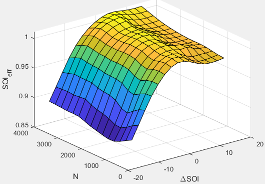Intake manifold gas pressure efficiency multiplier

CI Controller

The intake manifold gas pressure efficiency multiplier lookup table, ƒMAPeff, is a function of the intake manifold gas pressure ratio relative to optimal pressure ratio and lambda, MAPeff = ƒMAPeff(MAPratio,λ), where:

• MAPeff is intake manifold gas pressure efficiency multiplier, dimensionless.

• MAPratio is intake manifold gas pressure ratio relative to optimal pressure ratio, dimensionless.

• λ is intake manifold gas lambda, dimensionless.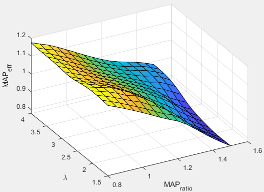Intake manifold gas temperature efficiency multiplier

CI Controller

The intake manifold gas temperature efficiency multiplier lookup table, ƒMATeff, is a function of the engine speed and intake manifold gas temperature relative to optimal temperature, MATeff = ƒMATeff(ΔMAT,N), where:

• MATeff is intake manifold gas temperature efficiency multiplier, dimensionless.

• ΔMAT is intake manifold gas temperature relative to optimal temperature, in K.

• N is engine speed, in rpm.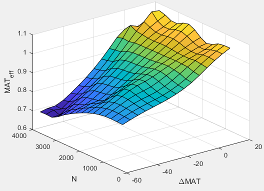Intake manifold gas oxygen efficiency multiplier

CI Controller

The intake manifold gas oxygen efficiency multiplier lookup table, ƒO2Peff, is a function of the engine speed and intake manifold gas oxygen percent relative to optimal, O2Peff = ƒO2Peff(ΔO2P,N), where:

• O2Peff is intake manifold gas oxygen efficiency multiplier, dimensionless.

• ΔO2P is intake gas oxygen percent relative to optimal, in percent.

• N is engine speed, in rpm.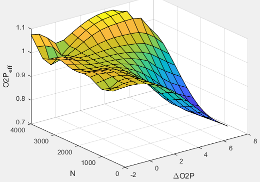Indicated mean effective pressure post inject correction

CI Controller

The indicated mean effective pressure post inject correction lookup table, ƒIMEPpost, is a function of the engine speed and fuel rail pressure relative to optimal breakpoints, ΔIMEPpost = ƒIMEPpost(ΔSOIpost,Fpost), where:

• ΔIMEPpost is indicated mean effective pressure post inject correction, in Pa.

• ΔSOIpost is indicated mean effective pressure post inject start of inject timing centroid, in degATDC.

• Fpost is indicated mean effective pressure post inject mass sum, in mg per injection.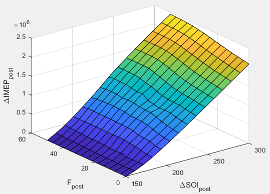Fuel rail pressure efficiency multiplier

CI Controller

The fuel rail pressure efficiency multiplier lookup table, ƒFUELPeff, is a function of the engine speed and fuel rail pressure relative to optimal breakpoints, FUELPeff = ƒFUELPeff(ΔFUELP,N), where:

• FUELPeff is fuel rail pressure efficiency multiplier, dimensionless.

• ΔFUELP is fuel rail pressure relative to optimal, in MPa.

• N is engine speed, in rpm.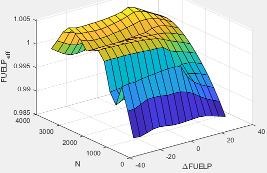Engine brake torque

CI Engine Simple Torque Model

CI Controller

For the simple torque lookup table model, the CI engine uses a lookup table is a function of engine speed and injected fuel mass, ${T}_{brake}={f}_{Tnf}\left(F,N\right)$, where:

• Tq = Tbrake is engine brake torque after accounting for engine mechanical and pumping friction effects, in N·m.

• F is injected fuel mass, in mg per injection.

• N is engine speed, in rpm.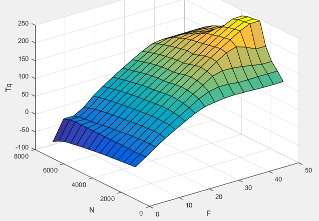Hydrocarbon (HC) mass fraction

HC emissions

CI Core Engine

The CI Core Engine HC emission mass fraction lookup table is a function of engine torque and engine speed, HC Mass Fraction = ƒ(Speed, Torque), where:

• HC Mass Fraction is the HC emission mass fraction, dimensionless.

• Speed is engine speed, in rpm.

• Torque is engine torque, in N·m.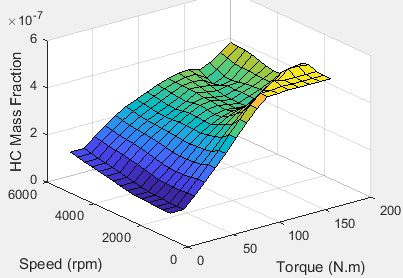Carbon monoxide (CO) mass fraction

CO emissions

CI Core Engine

The CI Core Engine CO emission mass fraction lookup table is a function of engine torque and engine speed, CO Mass Fraction = ƒ(Speed, Torque), where:

• CO Mass Fraction is the CO emission mass fraction, dimensionless.

• Speed is engine speed, in rpm.

• Torque is engine torque, in N·m.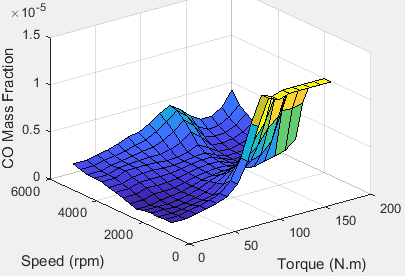Nitric oxide and nitrogen dioxide (NOx) mass fraction

NOx emissions

CI Core Engine

The CI Core Engine NOx emission mass fraction lookup table is a function of engine torque and engine speed, NOx Mass Fraction = ƒ(Speed, Torque), where:

• NOx Mass Fraction is the NOx emission mass fraction, dimensionless.

• Speed is engine speed, in rpm.

• Torque is engine torque, in N·m.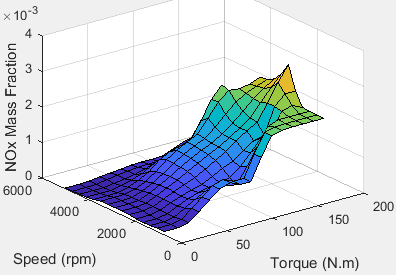Carbon dioxide (CO2) mass fraction

CO2 emissions

CI Core Engine

The CI Core Engine CO2 emission mass fraction lookup table is a function of engine torque and engine speed, CO2 Mass Fraction = ƒ(Speed, Torque), where:

• CO2 Mass Fraction is the CO2 emission mass fraction, dimensionless.

• Speed is engine speed, in rpm.

• Torque is engine torque, in N·m.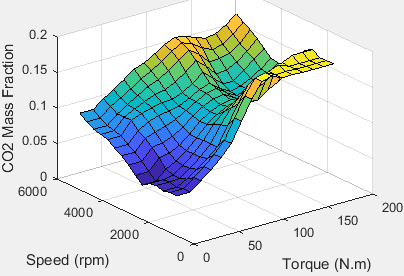Exhaust temperature

Engine exhaust temperature as a function of injected fuel mass and engine speed

CI Core Engine

CI Controller

The lookup table for the exhaust temperature is a function of injected fuel mass and engine speed

`${T}_{exh}={f}_{Texh}\left(F,N\right)$`

where:

• ${T}_{exh}$ is exhaust temperature, in K.

• F is injected fuel mass, in mg per injection.

• N is engine speed, in rpm.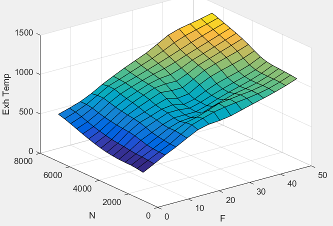Engine brake torque

Engine brake torque as a function of commanded fuel mass and engine speed

Mapped CI Engine

The engine brake torque lookup table is a function of commanded fuel mass and engine speed, ${T}_{brake}$ = ƒ(F, N), where:

• ${T}_{brake}$ is engine torque, in N·m.

• F is commanded fuel mass, in mg per injection.

• N is engine speed, in rpm.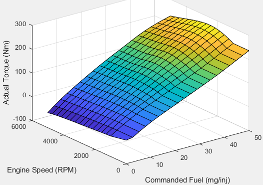Engine air mass flow

Engine air mass flow as a function of commanded fuel mass and engine speed

Mapped CI Engine

The air mass flow lookup table is a function of commanded fuel mass and engine speed, ${\stackrel{˙}{m}}_{intk}$ = ƒ(Fmax, N), where:

• ${\stackrel{˙}{m}}_{intk}$ is engine air mass flow, in kg/s.

• Fmax is commanded fuel mass, in mg per injection.

• N is engine speed, in rpm.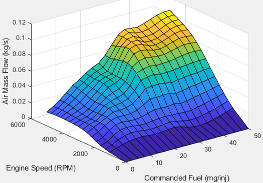Engine fuel flow

Engine fuel flow as a function of commanded fuel mass and engine speed

Mapped CI Engine

The engine fuel flow lookup table is a function of commanded fuel mass and engine speed, MassFlow= ƒ(F, N), where:

• MassFlow is engine fuel mass flow, in kg/s.

• F is commanded fuel mass, in mg per injection.

• N is engine speed, in rpm.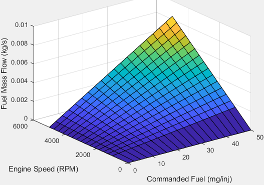Engine exhaust temperature

Engine exhaust temperature as a function of commanded fuel mass and engine speed

Mapped CI Engine

The engine exhaust temperature table is a function of commanded fuel mass and engine speed, Texh= ƒ(F, N), where:

• Texhis exhaust temperature, in K.

• F is commanded fuel mass, in mg per injection.

• N is engine speed, in rpm.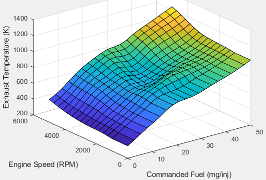Brake-specific fuel consumption (BSFC) efficiency

BSFC efficiency as a function of commanded fuel mass and engine speed

Mapped CI Engine

The brake-specific fuel consumption (BSFC) efficiency is a function of commanded fuel mass and engine speed, BSFC= ƒ(F, N), where:

• BSFC is BSFC, in g/kWh.

• F is commanded fuel mass, in mg per injection.

• N is engine speed, in rpm.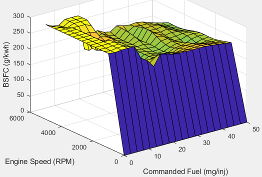Engine-out (EO) hydrocarbon emissions

EO hydrocarbon emissions as a function of commanded fuel mass and engine speed

Mapped CI Engine

The engine-out hydrocarbon emissions are a function of commanded fuel mass and engine speed, EO HC= ƒ(F, N), where:

• EO HC is engine-out hydrocarbon emissions, in kg/s.

• F is commanded fuel mass, in mg per injection.

• N is engine speed, in rpm.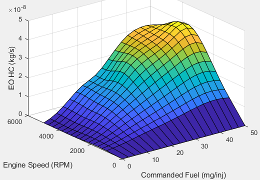Engine-out (EO) carbon monoxide emissions

EO carbon monoxide emissions as a function of commanded fuel mass and engine speed

Mapped CI Engine

The engine-out carbon monoxide emissions are a function of commanded fuel mass and engine speed, EO CO= ƒ(F, N), where:

• EO CO is engine-out carbon monoxide emissions, in kg/s.

• F is commanded fuel mass, in mg per injection.

• N is engine speed, in rpm.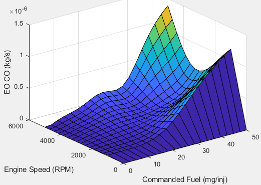Engine-out (EO) nitric oxide and nitrogen dioxide

EO nitric oxide and nitrogen dioxide emissions as a function of commanded fuel mass and engine speed

Mapped CI Engine

The engine-out nitric oxide and nitrogen dioxide emissions are a function of commanded fuel mass and engine speed, EO NOx= ƒ(F, N), where:

• EO NOx is engine-out nitric oxide and nitrogen dioxide emissions, in kg/s.

• F is commanded fuel mass, in mg per injection.

• N is engine speed, in rpm.Engine-out (EO) carbon dioxide emissions

EO carbon dioxide emissions as a function of commanded fuel mass and engine speed

Mapped CI Engine

The engine-out carbon dioxide emissions are a function of commanded fuel mass and engine speed, EO CO2= ƒ(F, N), where:

• EO CO2 is engine-out carbon dioxide emissions, in kg/s.

• F is commanded fuel mass, in mg per injection.

• N is engine speed, in rpm.Commanded exhaust gas recirculation (EGR) valve area percent

Commanded exhaust gas recirculation (EGR) valve area percent as a function of commanded torque and engine speed

CI Controller

The commanded exhaust gas recirculation (EGR) valve area percent lookup table is a function of commanded torque and engine speed

`$EG{R}_{cmd}={f}_{EGRcmd}\left(Tr{q}_{cmd},N\right)$`

where:

• EGRcmd is commanded EGR valve area percent, in percent.

• Trqcmd is commanded engine torque, in N·m.

• N is engine speed, in rpm.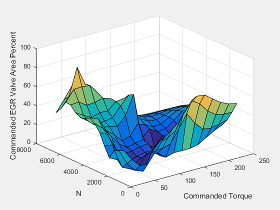Variable geometry turbocharger (VGT) rack position

Variable geometry turbocharger (VGT) rack position as a function of commanded torque and engine speed

CI Controller

The variable geometry turbocharger (VGT) rack position lookup table is a function of commanded torque and engine speed

`$R{P}_{cmd}={f}_{RPcmd}\left(Tr{q}_{cmd},N\right)$`

where:

• RPcmd is VGT rack position command, in percent.

• Trqcmd is commanded engine torque, in N·m.

• N is engine speed, in rpm.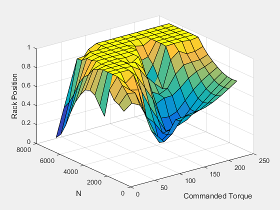Commanded total fuel mass per injection

Commanded total fuel mass per injection as a function of torque command and engine speed

CI Controller

The commanded total fuel mass per injection table is a function of the torque command and engine speed

`${F}_{cmd,tot}={f}_{Fcmd,tot}\left(Tr{q}_{cmd},N\right)$`

where:

• Fcmd,tot = F is commanded total fuel mass per injection, in mg per cylinder.

• Trqcmd is commanded engine torque, in N·m.

• N is engine speed, in rpm.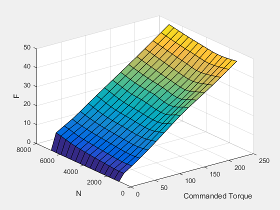Main start-of-injection (SOI) timing

SOI timing as a function of commanded fuel mass and engine speed

CI Controller

The main start-of-injection (SOI) timing lookup table is a function of commanded fuel mass and engine speed

`$MAINSOI=f\left({F}_{cmd,tot},N\right)$`

where:

• MAINSOI is the main start-of-injection timing, in degrees crank angle after top dead center (degATDC).

• Fcmd,tot = F is commanded fuel mass, in mg per injection.

• N is engine speed, in rpm.Standard exhaust gas recirculation (EGR) mass flow

EGR mass flow as a function of the standard flow pressure ratio and EGR valve flow area

CI Controller

The standard exhaust gas recirculation (EGR) mass flow is a lookup table that is a function of the standard flow pressure ratio and EGR valve flow area

`${\stackrel{˙}{m}}_{egr,std}=f\left(\frac{MAP}{{P}_{exh,est}},EGRap\right)$`

where:

• ${\stackrel{˙}{m}}_{egr,std}$ is the standard EGR valve mass flow, in g/s.

• Pexh,est is the estimated exhaust back-pressure, in Pa.

• MAP is the cycle average intake manifold absolute pressure, in Pa.

• EGRap is the measured EGR valve area, in percent.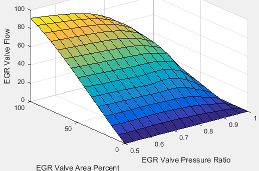Turbocharger pressure ratio

Turbocharger pressure ratio as a function of the standard air mass flow and corrected turbocharger speed

CI Controller

The turbocharger pressure ratio, corrected for variable geometry turbocharger (VGT) speed, is a lookup table that is a function of the standard air mass flow and corrected turbocharger speed, $P{r}_{turbo}=f\left({\stackrel{˙}{m}}_{airstd},{N}_{vgtcorr}\right)$, where:

• Prturbo is the turbocharger pressure ratio, corrected for VGT speed.

• ${\stackrel{˙}{m}}_{airstd}$ is the standard air mass flow, in g/s.

• Nvgtcorr is the corrected turbocharger speed, in rpm/K^(1/2).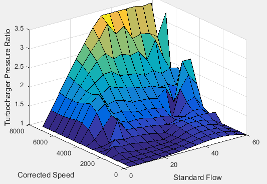Turbocharger pressure ratio correction

Turbocharger pressure ratio correction as a function of the rack position

CI Controller

The variable geometry turbocharger pressure ratio correction is a function of the rack position, Prvgtcorr= ƒ(VGTpos), where:

• Prvgtcorr is the turbocharger pressure ratio correction.

• VGTpos is the variable geometry turbocharger (VGT) rack position.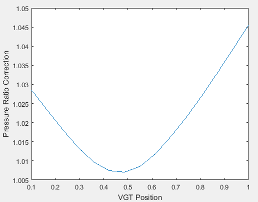### Calibration Maps in Spark-Ignition (SI) Blocks

In the engine models, the Powertrain Blockset blocks implement these calibration maps.

MapUsed forInDescription

Engine volumetric efficiency

SI Engine Speed-Density Air Mass Flow Model

SI Core Engine

SI Controller

The engine volumetric efficiency lookup table, ${f}_{{\eta }_{v}}$, is a function of intake manifold absolute pressure and engine speed

`${\eta }_{v}={f}_{{\eta }_{v}}\left(MAP,N\right)$`

where:

• ${\eta }_{v}$ is engine volumetric efficiency, dimensionless.

• MAP is intake manifold absolute pressure, in KPa.

• N is engine speed, in rpm.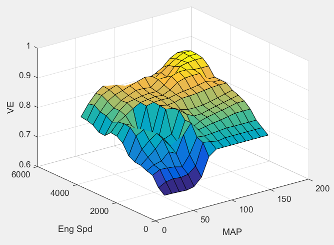Cylinder volume at intake valve close table (IVC)

SI Core Engine

SI Controller

The cylinder volume at intake valve close table (IVC), ${f}_{Vivc}$ is a function of the intake cam phaser angle

`${V}_{IVC}={f}_{Vivc}\left({\phi }_{ICP}\right)$`

where:

• ${V}_{IVC}$ is cylinder volume at IVC, in L.

• ${\phi }_{ICP}$ is intake cam phaser angle, in crank advance degrees.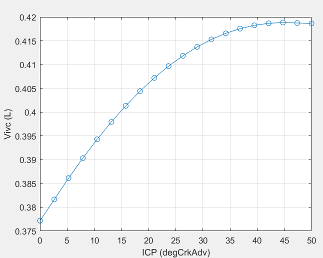Trapped mass correction

SI Core Engine

SI Controller

The trapped mass correction factor table, ${f}_{TMcorr}$, is a function of the normalized density and engine speed

where:

• $T{M}_{corr}$, is trapped mass correction multiplier, dimensionless.

• ${\rho }_{norm}$ is normalized density, dimensionless.

• N is engine speed, in rpm.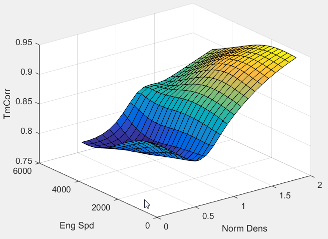Air mass flow at cam phaser angles

SI Core Engine

SI Controller

The phaser intake mass flow model lookup table is a function of exhaust cam phaser angles and trapped air mass flow

`${\stackrel{˙}{m}}_{intkideal}={f}_{intkideal}\left({\phi }_{ECP},T{M}_{flow}\right)$`

where:

• ${\stackrel{˙}{m}}_{intkideal}$ is engine intake port mass flow at arbitrary cam phaser angles, in g/s.

• ${\phi }_{ECP}$ is exhaust cam phaser angle, in degrees crank retard.

• $T{M}_{flow}$ is flow rate equivalent to corrected trapped mass at the current engine speed, in g/s.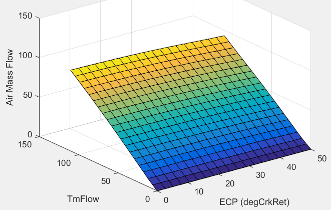Air mass flow correction

SI Core Engine

SI Controller

The intake air mass flow correction lookup table, ${f}_{aircorr}$, is a function of ideal load and engine speed

`${\stackrel{˙}{m}}_{air}={\stackrel{˙}{m}}_{intkideal}{f}_{aircorr}\left({L}_{ideal},N\right)$`

where:

• ${L}_{ideal}$ is engine load (normalized cylinder air mass) at arbitrary cam phaser angles, uncorrected for final steady-state cam phaser angles, dimensionless.

• N is engine speed, in rpm.

• ${\stackrel{˙}{m}}_{air}$ is engine intake air mass flow final correction at steady-state cam phaser angles, in g/s.

• ${\stackrel{˙}{m}}_{intkideal}$ is engine intake port mass flow at arbitrary cam phaser angles, in g/s.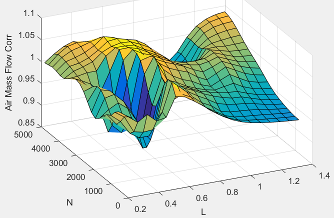Inner torque

SI Engine Torque Structure Model

SI Core Engine

SI Controller

The inner torque lookup table, ${f}_{Tqinr}$, is a function of engine speed and engine load, $T{q}_{inr}={f}_{Tqinr}\left(L,N\right)$, where:

• $T{q}_{inr}$ is inner torque based on gross indicated mean effective pressure, in N·m.

• L is engine load at arbitrary cam phaser angles, corrected for final steady-state cam phaser angles, dimensionless.

• N is engine speed, in rpm.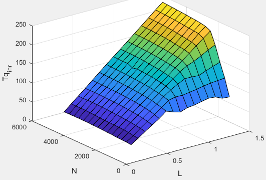Friction torque

SI Engine Torque Structure Model

SI Controller

The friction torque lookup table, ${f}_{Tfric}$, is a function of engine speed and engine load, ${T}_{fric}={f}_{Tfric}\left(L,N\right)$, where:

• ${T}_{fric}$ is friction torque offset to inner torque, in N·m.

• L is engine load at arbitrary cam phaser angles, corrected for final steady-state cam phaser angles, dimensionless.

• N is engine speed, in rpm.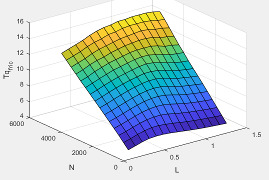Pumping torque

SI Engine Torque Structure Model

SI Controller

The pumping work lookup table, ƒTpump, is a function of engine load and engine speed, `Tpump=ƒTpump(L,N)`, where:

• Tpump is pumping work, in N·m.

• L is engine load, as a normalized cylinder air mass, dimensionless.

• N is engine speed, in rpm.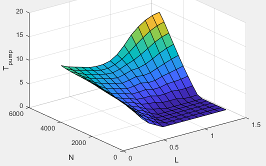SI Engine Torque Structure Model

SI Controller

The optimal spark lookup table, ${f}_{SAopt}$, is a function of engine speed and engine load, $S{A}_{opt}={f}_{SAopt}\left(L,N\right)$, where:

• SAopt is optimal spark advance timing for maximum inner torque at stoichiometric air-fuel ratio (AFR), in deg.

• L is engine load at arbitrary cam phaser angles, corrected for final steady-state cam phaser angles, dimensionless.

• N is engine speed, in rpm.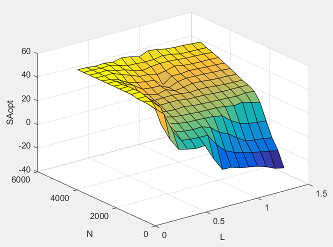Spark efficiency

SI Engine Torque Structure Model

SI Controller

The spark efficiency lookup table, ${f}_{Msa}$, is a function of the spark retard from optimal

`$\begin{array}{l}{M}_{sa}={f}_{Msa}\left(\Delta SA\right)\\ \Delta SA=S{A}_{opt}-SA\end{array}$`

where:

• ${M}_{sa}$ is the spark retard efficiency multiplier, dimensionless.

• $\Delta SA$is the spark retard timing distance from optimal spark advance, in deg.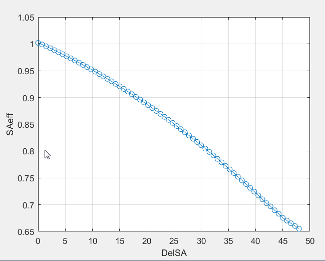Lambda efficiency

SI Engine Torque Structure Model

SI Controller

The lambda efficiency lookup table, ${f}_{M\lambda }$, is a function of lambda, ${M}_{\lambda }={f}_{M\lambda }\left(\lambda \right)$, where:

• ${M}_{\lambda }$ is the lambda multiplier on inner torque to account for the air-fuel ratio (AFR) effect, dimensionless.

• $\lambda$ is lambda, AFR normalized to stoichiometric fuel AFR, dimensionless.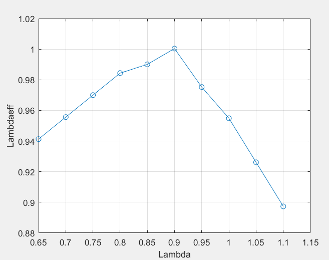Simple torque

SI Engine Simple Torque Model

SI Core Engine

SI Controller

For the simple torque lookup table model, the SI engine uses a lookup table map that is a function of engine speed and load, ${T}_{brake}={f}_{TnL}\left(L,N\right)$, where:

• ${T}_{brake}$ is engine brake torque after accounting for spark advance, AFR, and friction effects, in N·m.

• L is engine load, as a normalized cylinder air mass, dimensionless.

• N is engine speed, in rpm.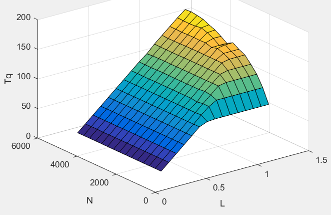Hydrocarbon (HC) mass fraction

HC emissions

SI Core Engine

The SI Core Engine HC emission mass fraction lookup table is a function of engine torque and engine speed, HC Mass Fraction = ƒ(Speed, Torque), where:

• HC Mass Fraction is the HC emission mass fraction, dimensionless.

• Speed is engine speed, in rpm.

• Torque is engine torque, in N·m.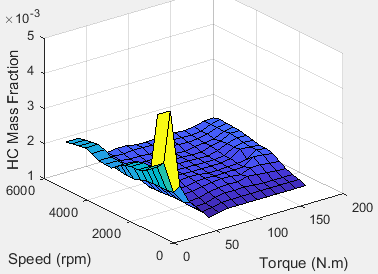Carbon monoxide (CO) mass fraction

CO emissions

SI Core Engine

The SI Core Engine CO emission mass fraction lookup table is a function of engine torque and engine speed, CO Mass Fraction = ƒ(Speed, Torque), where:

• CO Mass Fraction is the CO emission mass fraction, dimensionless.

• Speed is engine speed, in rpm.

• Torque is engine torque, in N·m.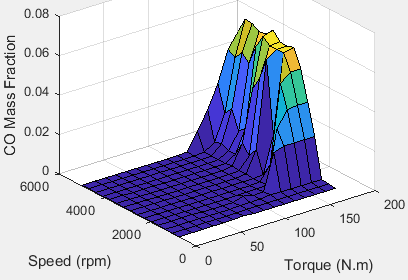Nitric oxide and nitrogen dioxide (NOx) mass fraction

NOx emissions

SI Core Engine

The SI Core Engine NOx emission mass fraction lookup table is a function of engine torque and engine speed, NOx Mass Fraction = ƒ(Speed, Torque), where:

• NOx Mass Fraction is the NOx emission mass fraction, dimensionless.

• Speed is engine speed, in rpm.

• Torque is engine torque, in N·m.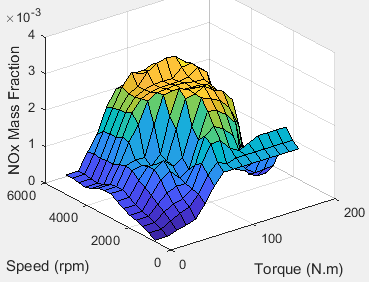Carbon dioxide (CO2) mass fraction

CO2 emissions

SI Core Engine

The SI Core Engine CO2 emission mass fraction lookup table is a function of engine torque and engine speed, CO2 Mass Fraction = ƒ(Speed, Torque), where:

• CO2 Mass Fraction is the CO2 emission mass fraction, dimensionless.

• Speed is engine speed, in rpm.

• Torque is engine torque, in N·m.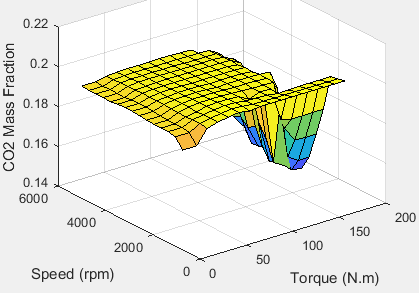Exhaust temperature

Engine exhaust calculation as a function of engine speed and load

SI Controller

The exhaust temperature lookup table, ${f}_{Texh}$, is a function of engine load and engine speed

`${T}_{exh}={f}_{Texh}\left(L,N\right)$`

where:

• Texh is engine exhaust temperature, in K.

• L is normalized cylinder air mass or engine load, dimensionless.

• N is engine speed, in rpm.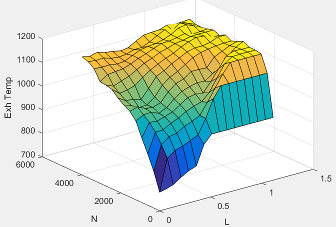Engine torque

Engine brake torque as a function of commanded torque and engine speed

Mapped SI Engine

The engine torque lookup table is a function of commanded engine torque and engine speed, T = ƒ(Tcmd, N), where:

• T is engine torque, in N·m.

• Tcmd is commanded engine torque, in N·m.

• N is engine speed, in rpm.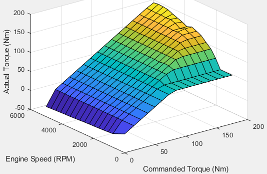Engine air mass flow

Engine air mass flow as a function of commanded torque and engine speed

Mapped SI Engine

The engine air mass flow lookup table is a function of commanded engine torque and engine speed, ${\stackrel{˙}{m}}_{intk}$ = ƒ(Tcmd, N), where:

• ${\stackrel{˙}{m}}_{intk}$ is engine air mass flow, in kg/s.

• Tcmd is commanded engine torque, in N·m.

• N is engine speed, in rpm.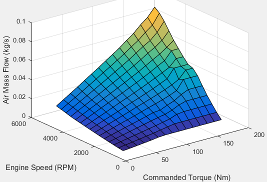Engine fuel flow

Engine fuel flow as a function of commanded torque mass and engine speed

Mapped SI Engine

The engine fuel mass flow lookup table is a function of commanded engine torque and engine speed, MassFlow = ƒ(Tcmd, N), where:

• MassFlow is engine fuel mass flow, in kg/s.

• Tcmd is commanded engine torque, in N·m.

• N is engine speed, in rpm.Engine exhaust temperature

Engine exhaust temperature as a function of commanded torque and engine speed

Mapped SI Engine

The engine exhaust temperature lookup table is a function of commanded engine torque and engine speed, Texh = ƒ(Tcmd, N), where:

• Texh is exhaust temperature, in K.

• Tcmd is commanded engine torque, in N·m.

• N is engine speed, in rpm.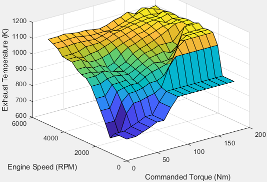Brake-specific fuel consumption (BSFC) efficiency

Brake-specific fuel consumption (BSFC) as a function of commanded torque and engine speed

Mapped SI Engine

The brake-specific fuel consumption (BSFC) efficiency is a function of commanded engine torque and engine speed, BSFC = ƒ(Tcmd, N), where:

• BSFC is BSFC, in g/kWh.

• Tcmd is commanded engine torque, in N·m.

• N is engine speed, in rpm.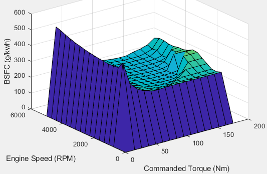Engine-out (EO) hydrocarbon emissions

EO hydrocarbon emissions as a function of commanded torque and engine speed

Mapped SI Engine

The engine-out hydrocarbon emissions are a function of commanded engine torque and engine speed, EO HC = ƒ(Tcmd, N), where:

• EO HC is engine-out hydrocarbon emissions, in kg/s.

• Tcmd is commanded engine torque, in N·m.

• N is engine speed, in rpm.Engine-out (EO) carbon monoxide emissions

EO carbon monoxide emissions as a function of commanded torque and engine speed

Mapped SI Engine

The engine-out carbon monoxide emissions are a function of commanded engine torque and engine speed, EO CO = ƒ(Tcmd, N), where:

• EO CO is engine-out carbon monoxide emissions, in kg/s.

• Tcmd is commanded engine torque, in N·m.

• N is engine speed, in rpm.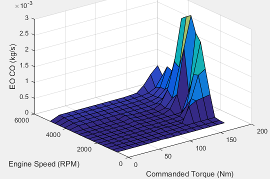Engine-out (EO) nitric oxide and nitrogen dioxide emissions

EO nitric oxide and nitrogen dioxide emissions as a function of commanded torque and engine speed

Mapped SI Engine

The engine-out nitric oxide and nitrogen dioxide emissions are a function of commanded engine torque and engine speed, EO NOx = ƒ(Tcmd, N), where:

• EO NOx is engine-out nitric oxide and nitrogen dioxide emissions, in kg/s.

• Tcmd is commanded engine torque, in N·m.

• N is engine speed, in rpm.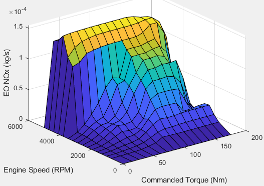Engine-out (EO) carbon dioxide emissions

EO carbon dioxide emissions as a function of commanded torque and engine speed

Mapped SI Engine

The engine-out carbon dioxide emissions are a function of commanded engine torque and engine speed, EO CO2 = ƒ(Tcmd, N), where:

• EO CO2 is engine-out carbon dioxide emissions, in kg/s.

• Tcmd is commanded engine torque, in N·m.

• N is engine speed, in rpm.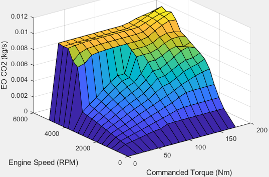Wastegate area percent command

Wastegate area percent command as a function of the commanded engine load and engine speed

SI Controller

The wastegate area percent command lookup table, ${f}_{WAPcmd}$, is a function of the commanded engine load and engine speed

`$WA{P}_{cmd}={f}_{WAPcmd}\left({L}_{cmd},N\right)$`

where:

• WAPcmd is wastegate area percentage command, in percent.

• Lcmd=L is commanded engine load, dimensionless.

• N is engine speed, in rpm.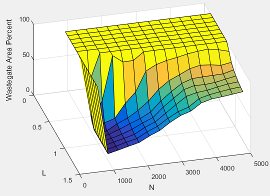Throttle position percent command

Throttle position percent command as a function of the throttle area percentage command

SI Controller

The throttle position percent command lookup table, ${f}_{TPPcmd}$, is a function of the throttle area percentage command

`$TP{P}_{cmd}={f}_{TPPcmd}\left(TA{P}_{cmd}\right)$`

where:

• TPPcmd is throttle position percentage command, in percent.

• TAPcmd is throttle area percentage command, in percent.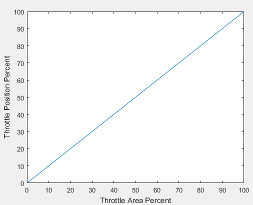Throttle area percent command

Throttle area percent command as a function of commanded load and engine speed

SI Controller

The throttle area percent command lookup table, ${f}_{TAPcmd}$, is a function of commanded load and engine speed

`$TA{P}_{cmd}={f}_{TAPcmd}\left({L}_{cmd},N\right)$`

where:

• TAPcmd is throttle area percentage command, in percent.

• Lcmd=L is commanded engine load, dimensionless.

• N is engine speed, in rpm.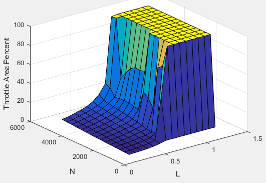SI Controller

The spark advance lookup table is a function of estimated load and engine speed.

`$SA={f}_{SA}\left({L}_{est},N\right)$`

where:

• Lest=L is estimated engine load, dimensionless.

• N is engine speed, in rpm.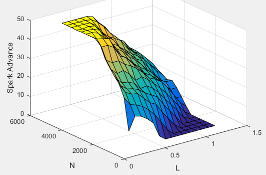Commanded lambda

Commanded lambda as a function of estimated engine load and measured engine speed

SI Controller

The commanded lambda, ${\lambda }_{cmd}$, lookup table is a function of estimated engine load and measured engine speed

`${\lambda }_{cmd}={f}_{\lambda cmd}\left({L}_{est},N\right)$`

where:

• ${\lambda }_{cmd}$ is commanded relative AFR, dimensionless.

• Lest=L is estimated engine load, dimensionless.

• N is engine speed, in rpm.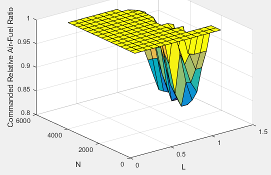Intake cam phaser angle command

Intake cam phaser angle command as a function of the engine load and engine speed

SI Controller

The intake cam phaser angle command lookup table, ${f}_{ICPCMD}$, is a function of the engine load and engine speed

`${\phi }_{ICPCMD}={f}_{ICPCMD}\left({L}_{est},N\right)$`

where:

• ${\phi }_{ICPCMD}$ is commanded intake cam phaser angle, in degrees crank advance.

• Lest=L is estimated engine load, dimensionless.

• N is engine speed, in rpm.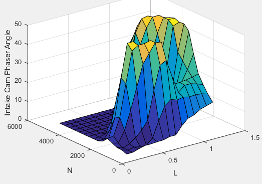Commanded engine load as a function of the commanded torque and engine speed

SI Controller

The commanded engine load lookup table, ${f}_{Lcmd}$, is a function of the commanded torque and engine speed

`${L}_{cmd}={f}_{Lcmd}\left({T}_{cmd},N\right)$`

where:

• Lcmd=L is commanded engine load, dimensionless.

• Tcmd is commanded torque, in N·m.

• N is engine speed, in rpm.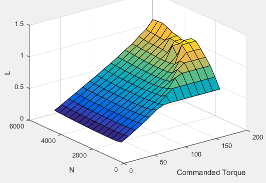Exhaust cam phaser angle

Exhaust cam phaser angle as a function of the engine load and engine speed

SI Controller

The exhaust cam phaser angle command lookup table, ${f}_{ECPCMD}$, is a function of the engine load and engine speed

`${\phi }_{ECPCMD}={f}_{ECPCMD}\left({L}_{est},N\right)$`

where:

• ${\phi }_{ECPCMD}$ is commanded exhaust cam phaser angle, in degrees crank retard.

• Lest=L is estimated engine load, dimensionless.

• N is engine speed, in rpm.